# Category: noncommutative

For some time I knew it was in the making, now they are ready to launch it:

The $\mathbb{F}_1$ World Seminar, an online seminar dedicated to the “field with one element”, and its many connections to areas in mathematics such as arithmetic, geometry, representation theory and combinatorics. The organisers are Jaiung Jun, Oliver Lorscheid, Yuri Manin, Matt Szczesny, Koen Thas and Matt Young.

From the announcement:

“While the origins of the “$\mathbb{F}_1$-story” go back to attempts to transfer Weil’s proof of the Riemann Hypothesis from the function field case to that of number fields on one hand, and Tits’s Dream of realizing Weyl groups as the $\mathbb{F}_1$ points of algebraic groups on the other, the “$\mathbb{F}_1$” moniker has come to encompass a wide variety of phenomena and analogies spanning algebraic geometry, algebraic topology, arithmetic, combinatorics, representation theory, non-commutative geometry etc. It is therefore impossible to compile an exhaustive list of topics that might be discussed. The following is but a small sample of topics that may be covered:

Algebraic geometry in non-additive contexts – monoid schemes, lambda-schemes, blue schemes, semiring and hyperfield schemes, etc.
Arithmetic – connections with motives, non-archimedean and analytic geometry
Tropical geometry and geometric matroid theory
Algebraic topology – K-theory of monoid and other “non-additive” schemes/categories, higher Segal spaces
Representation theory – Hall algebras, degenerations of quantum groups, quivers
Combinatorics – finite field and incidence geometry, and various generalizations”

The seminar takes place on alternating Wednesdays from 15:00 PM – 16:00 PM European Standard Time (=GMT+1). There will be room for mathematical discussion after each lecture.

The first meeting takes place Wednesday, January 19th 2022. If you want to receive abstracts of the talks and their Zoom-links, you should sign up for the mailing list.

Perhaps I’ll start posting about $\mathbb{F}_1$ again, either here, or on the dormant $\mathbb{F}_1$ mathematics blog. (see this post for its history).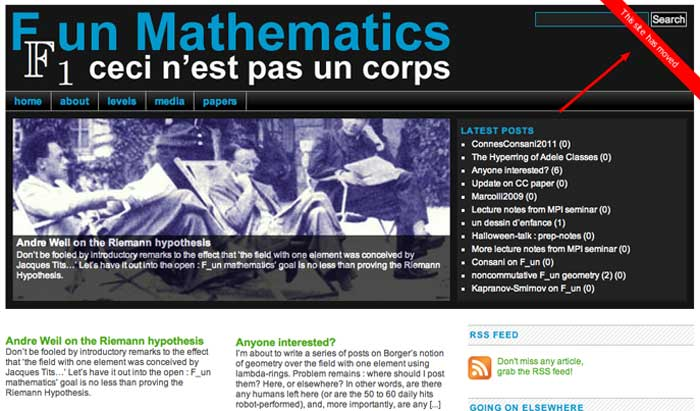Last time I mentioned the talk “From noncommutative geometry to the tropical geometry of the scaling site” by Alain Connes, culminating in the canonical isomorphism (last slide of the talk)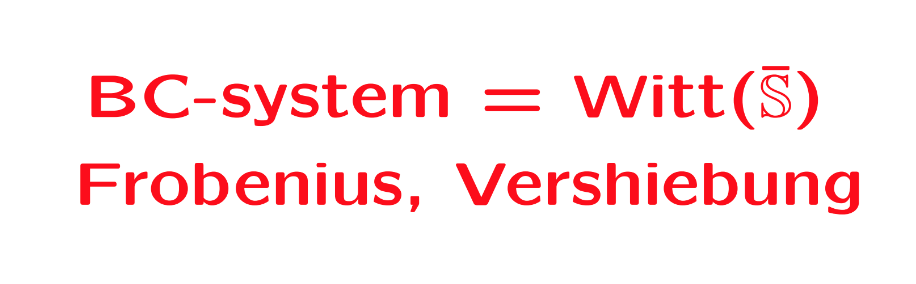Or rather, what is actually proved in his paper with Caterina Consani BC-system, absolute cyclotomy and the quantized calculus (and which they conjectured previously to be the case in Segal’s Gamma rings and universal arithmetic), is a canonical isomorphism between the $\lambda$-rings
$\mathbb{Z}[\mathbb{Q}/\mathbb{Z}] \simeq \mathbb{W}_0(\overline{\mathbb{S}})$
The left hand side is the integral groupring of the additive quotient-group $\mathbb{Q}/\mathbb{Z}$, or if you prefer, $\mathbb{Z}[\mathbf{\mu}_{\infty}]$ the integral groupring of the multiplicative group of all roots of unity $\mathbf{\mu}_{\infty}$.

The power maps on $\mathbf{\mu}_{\infty}$ equip $\mathbb{Z}[\mathbf{\mu}_{\infty}]$ with a $\lambda$-ring structure, that is, a family of commuting endomorphisms $\sigma_n$ with $\sigma_n(\zeta) = \zeta^n$ for all $\zeta \in \mathbf{\mu}_{\infty}$, and a family of linear maps $\rho_n$ induced by requiring for all $\zeta \in \mathbf{\mu}_{\infty}$ that
$\rho_n(\zeta) = \sum_{\mu^n=\zeta} \mu$
The maps $\sigma_n$ and $\rho_n$ are used to construct an integral version of the Bost-Connes algebra describing the Bost-Connes sytem, a quantum statistical dynamical system.

On the right hand side, $\mathbb{S}$ is the sphere spectrum (an object from stable homotopy theory) and $\overline{\mathbb{S}}$ its ‘algebraic closure’, that is, adding all abstract roots of unity.

The ring $\mathbb{W}_0(\overline{\mathbb{S}})$ is a generalisation to the world of spectra of the Almkvist-ring $\mathbb{W}_0(R)$ defined for any commutative ring $R$, constructed from pairs $(E,f)$ where $E$ is a projective $R$-module of finite rank and $f$ an $R$-endomorphism on it. Addition and multiplication are coming from direct sums and tensor products of such pairs, with zero element the pair $(0,0)$ and unit element the pair $(R,1_R)$. The ring $\mathbb{W}_0(R)$ is then the quotient-ring obtained by dividing out the ideal consisting of all zero-pairs $(E,0)$.

The ring $\mathbb{W}_0(R)$ becomes a $\lambda$-ring via the Frobenius endomorphisms $F_n$ sending a pair $(E,f)$ to the pair $(E,f^n)$, and we also have a collection of linear maps on $\mathbb{W}_0(R)$, the ‘Verschiebung’-maps which send a pair $(E,f)$ to the pair $(E^{\oplus n},F)$ with
$F = \begin{bmatrix} 0 & 0 & 0 & \cdots & f \\ 1 & 0 & 0 & \cdots & 0 \\ 0 & 1 & 0 & \cdots & 0 \\ \vdots & \vdots & \vdots & & \vdots \\ 0 & 0 & 0 & \cdots & 1 \end{bmatrix}$
Connes and Consani define a notion of modules and their endomorphisms for $\mathbb{S}$ and $\overline{\mathbb{S}}$, allowing them to define in a similar way the rings $\mathbb{W}_0(\mathbb{S})$ and $\mathbb{W}_0(\overline{\mathbb{S}})$, with corresponding maps $F_n$ and $V_n$. They then establish an isomorphism with $\mathbb{Z}[\mathbb{Q}/\mathbb{Z}]$ such that the maps $(F_n,V_n)$ correspond to $(\sigma_n,\rho_n)$.

But, do we really have the go to spectra to achieve this?

All this reminds me of an old idea of Yuri Manin mentioned in the introduction of his paper Cyclotomy and analytic geometry over $\mathbb{F}_1$, and later elaborated in section two of his paper with Matilde Marcolli Homotopy types and geometries below $\mathbf{Spec}(\mathbb{Z})$.

Take a manifold $M$ with a diffeomorphism $f$ and consider the corresponding discrete dynamical system by iterating the diffeomorphism. In such situations it is important to investigate the periodic orbits, or the fix-points $Fix(M,f^n)$ for all $n$. If we are in a situation that the number of fixed points is finite we can package these numbers in the Artin-Mazur zeta function
$\zeta_{AM}(M,f) = exp(\sum_{n=1}^{\infty} \frac{\# Fix(M,f^n)}{n}t^n)$
and investigate the properties of this function.

To connect this type of problem to Almkvist-like rings, Manin considers the Morse-Smale dynamical systems, a structural stable diffeomorphism $f$, having a finite number of non-wandering points on a compact manifold $M$.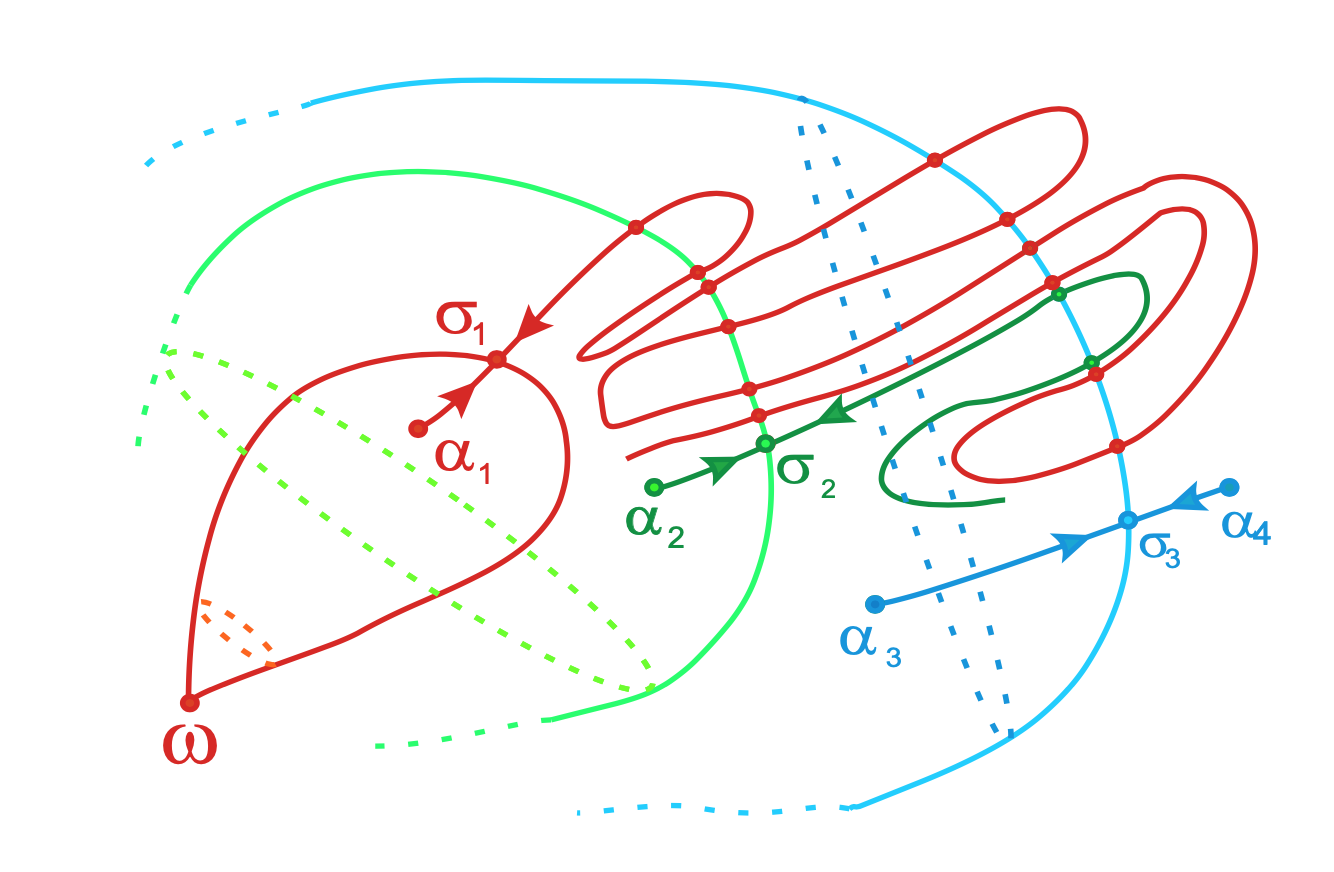From Topological classification of Morse-Smale diffeomorphisms on 3-manifolds

In such a situation $f_{\ast}$ acts on homology $H_k(M,\mathbb{Z})$, which are free $\mathbb{Z}$-modules of finite rank, as a matrix $M_f$ having only roots of unity as its eigenvalues.

Manin argues that this action is similar to the action of the Frobenius on etale cohomology groups, in which case the eigenvalues are Weil numbers. That is, one might view roots of unity as Weil numbers in characteristic one.

Clearly, all relevant data $(H_k(M,\mathbb{Z}),f_{\ast})$ belongs to the $\lambda$-subring of $\mathbb{W}_0(\mathbb{Z})$ generated by all pairs $(E,f)$ such that $M_f$ is diagonalisable and all its eigenvalues are either $0$ or roots of unity.

If we denote for any ring $R$ by $\mathbb{W}_1(R)$ this $\lambda$-subring of $\mathbb{W}_0(R)$, probably one would obtain canonical isomorphisms

– between $\mathbb{W}_1(\mathbb{Z})$ and the invariant part of the integral groupring $\mathbb{Z}[\mathbb{Q}/\mathbb{Z}]$ for the action of the group $Aut(\mathbb{Q}/\mathbb{Z}) = \widehat{\mathbb{Z}}^*$, and

– between $\mathbb{Z}[\mathbb{Q}/\mathbb{Z}]$ and $\mathbb{W}_1(\mathbb{Z}(\mathbf{\mu}_{\infty}))$ where $\mathbb{Z}(\mathbf{\mu}_{\infty})$ is the ring obtained by adjoining to $\mathbb{Z}$ all roots of unity.

In recent months, my primary focus was on teaching and family matters, so I make advantage of this Christmas break to catch up with some of the things I’ve missed.

Peter Woit’s blog alerted me to the existence of the (virtual) Lake Como-conference, end of september: Unifying themes in Geometry.

In Corona times, virtual conferences seem to sprout up out of nowhere, everywhere (zero costs), giving us an inflation of YouTubeD talks. I’m always grateful to the organisers of such events to provide the slides of the talks separately, as the generic YouTubeD-talk consists merely in reading off the slides.

Allow me to point you to one of the rare exceptions to this rule.

When I downloaded the slides of Alain Connes’ talk at the conference From noncommutative geometry to the tropical geometry of the scaling site I just saw a collage of graphics from his endless stream of papers with Katia Consani, and slides I’d seen before watching several of his YouTubeD-talks in recent years.

Boy, am I glad I gave Alain 5 minutes to convince me this talk was different.

For the better part of his talk, Alain didn’t just read off the slides, but rather tried to explain the thought processes that led him and Katia to move on from the results on this slide to those on the next one.

If you’re pressed for time, perhaps you might join in at 49.34 into the talk, when he acknowledges the previous (tropical) approach ran out of steam as they were unable to define any $H^1$ properly, and how this led them to ‘absolute’ algebraic geometry, meaning over the sphere spectrum $\mathbb{S}$.

Sadly, for some reason Alain didn’t manage to get his final two slides on screen. So, in this case, the slides actually add value to the talk…

A Belyi-extender (or dessinflateur) $\beta$ of degree $d$ is a quotient of two polynomials with rational coefficients
$\beta(t) = \frac{f(t)}{g(t)}$
with the special properties that for each complex number $c$ the polynomial equation of degree $d$ in $t$
$f(t)-c g(t)=0$
has $d$ distinct solutions, except perhaps for $c=0$ or $c=1$, and, in addition, we have that
$\beta(0),\beta(1),\beta(\infty) \in \{ 0,1,\infty \}$

Let’s take for instance the power maps $\beta_n(t)=t^n$.

For every $c$ the degree $n$ polynomial $t^n – c = 0$ has exactly $n$ distinct solutions, except for $c=0$, when there is just one. And, clearly we have that $0^n=0$, $1^n=1$ and $\infty^n=\infty$. So, $\beta_n$ is a Belyi-extender of degree $n$.

A cute observation being that if $\beta$ is a Belyi-extender of degree $d$, and $\beta’$ is an extender of degree $d’$, then $\beta \circ \beta’$ is again a Belyi-extender, this time of degree $d.d’$.

That is, Belyi-extenders form a monoid under composition!

In our example, $\beta_n \circ \beta_m = \beta_{n.m}$. So, the power-maps are a sub-monoid of the Belyi-extenders, isomorphic to the multiplicative monoid $\mathbb{N}_{\times}$ of strictly positive natural numbers.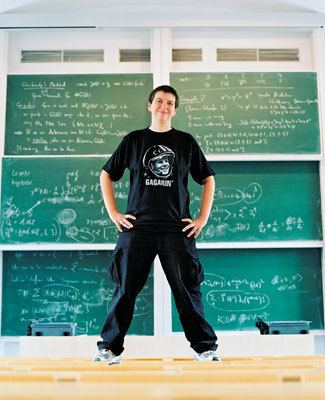In their paper Quantum statistical mechanics of the absolute Galois group, Yuri I. Manin and Matilde Marcolli say they use the full monoid of Belyi-extenders to act on all Grothendieck’s dessins d’enfant.

But, they attach properties to these Belyi-extenders which they don’t have, in general. That’s fine, as they foresee in Remark 2.21 of their paper that the construction works equally well for any suitable sub-monoid, as long as this sub-monoid contains all power-map exenders.

I’m trying to figure out what the maximal mystery sub-monoid of extenders is satisfying all the properties they need for their proofs.

But first, let us see what Belyi-extenders have to do with dessins d’enfant.In his user-friendlier period, Grothendieck told us how to draw a picture, which he called a dessin d’enfant, of an extender $\beta(t) = \frac{f(t)}{g(t)}$ of degree $d$:

Look at all complex solutions of $f(t)=0$ and label them with a black dot (and add a black dot at $\infty$ if $\beta(\infty)=0$). Now, look at all complex solutions of $f(t)-g(t)=0$ and label them with a white dot (and add a white dot at $\infty$ if $\beta(\infty)=1$).

Now comes the fun part.

Because $\beta$ has exactly $d$ pre-images for all real numbers $\lambda$ in the open interval $(0,1)$ (and $\beta$ is continuous), we can connect the black dots with the white dots by $d$ edges (the pre-images of the open interval $(0,1)$), giving us a $2$-coloured graph.

For the power-maps $\beta_n(t)=t^n$, we have just one black dot at $0$ (being the only solution of $t^n=0$), and $n$ white dots at the $n$-th roots of unity (the solutions of $x^n-1=0$). Any $\lambda \in (0,1)$ has as its $n$ pre-images the numbers $\zeta_i.\sqrt[n]{\lambda}$ with $\zeta_i$ an $n$-th root of unity, so we get here as picture an $n$-star. Here for $n=5$: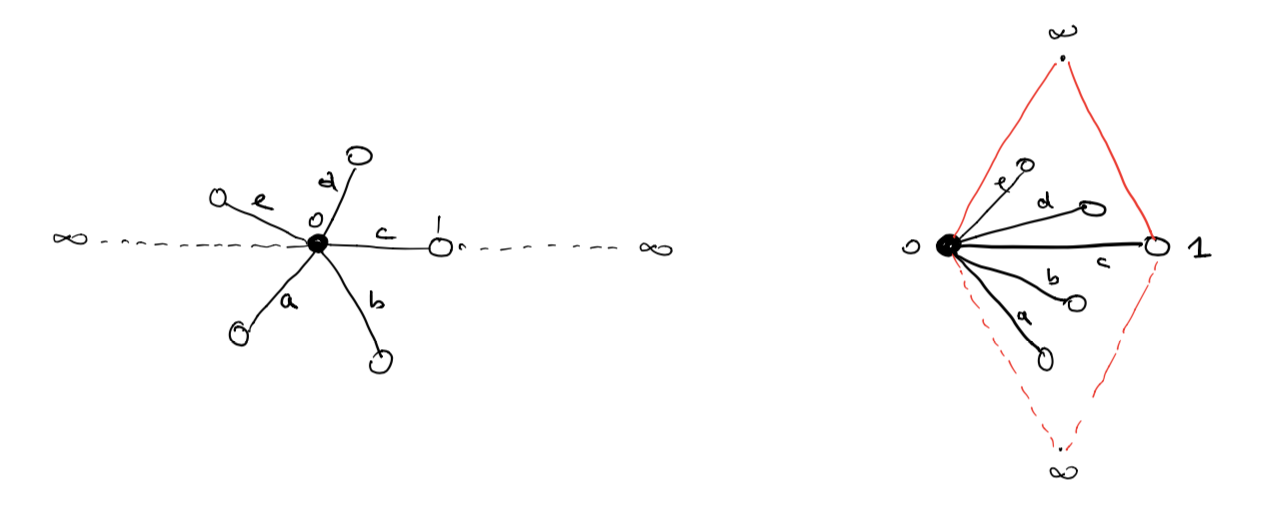This dessin should be viewed on the 2-sphere, with the antipodal point of $0$ being $\infty$, so projecting from $\infty$ gives a homeomorphism between the 2-sphere and $\mathbb{C} \cup \{ \infty \}$.

To get all information of the dessin (including possible dots at infinity) it is best to slice the sphere open along the real segments $(\infty,0)$ and $(1,\infty)$ and flatten it to form a ‘diamond’ with the upper triangle corresponding to the closed upper semisphere and the lower triangle to the open lower semisphere.

In the picture above, the right hand side is the dessin drawn in the diamond, and this representation will be important when we come to the action of extenders on more general Grothendieck dessins d’enfant.

Okay, let’s try to get some information about the monoid $\mathcal{E}$ of all Belyi-extenders.

What are its invertible elements?

Well, we’ve seen that the degree of a composition of two extenders is the product of their degrees, so invertible elements must have degree $1$, so are automorphisms of $\mathbb{P}^1_{\mathbb{C}} – \{ 0,1,\infty \} = S^2-\{ 0,1,\infty \}$ permuting the set $\{ 0,1,\infty \}$.

They form the symmetric group $S_3$ on $3$-letters and correspond to the Belyi-extenders
$t,~1-t,~\frac{1}{t},~\frac{1}{1-t},~\frac{t-1}{t},~\frac{t}{t-1}$
You can compose these units with an extender to get anther extender of the same degree where the roles of $0,1$ and $\infty$ are changed.

For example, if you want to colour all your white dots black and the black dots white, you compose with the unit $1-t$.

Manin and Marcolli use this and claim that you can transform any extender $\eta$ to an extender $\gamma$ by composing with a unit, such that $\gamma(0)=0, \gamma(1)=1$ and $\gamma(\infty)=\infty$.

That’s fine as long as your original extender $\eta$ maps $\{ 0,1,\infty \}$ onto $\{ 0,1,\infty \}$, but usually a Belyi-extender only maps into $\{ 0,1,\infty \}$.

Here are some extenders of degree three (taken from Melanie Wood’s paper Belyi-extending maps and the Galois action on dessins d’enfants):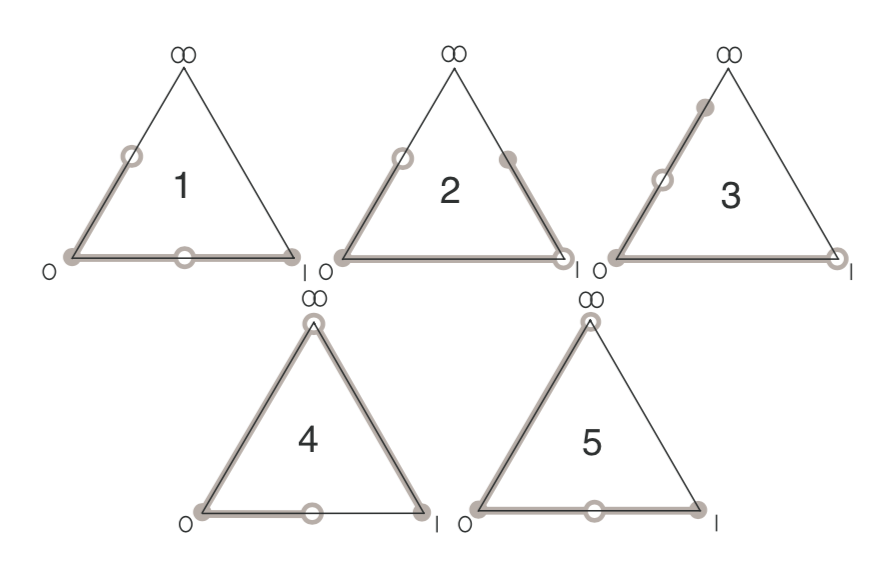with dessin $5$ corresponding to the Belyi-extender
$\beta(t) = \frac{t^2(t-1)}{(t-\frac{4}{3})^3}$
with $\beta(0)=0=\beta(1)$ and $\beta(\infty) = 1$.

So, a first property of the mystery Manin-Marcolli monoid $\mathcal{E}_{MMM}$ must surely be that all its elements $\gamma(t)$ map $\{ 0,1,\infty \}$ onto $\{ 0,1,\infty \}$, for they use this property a number of times, for instance to construct a monoid map
$\mathcal{E}_{MMM} \rightarrow M_2(\mathbb{Z})^+ \qquad \gamma \mapsto \begin{bmatrix} d & m-1 \\ 0 & 1 \end{bmatrix}$
where $d$ is the degree of $\gamma$ and $m$ is the number of black dots in the dessin (or white dots for that matter).

Further, they seem to believe that the dessin of any Belyi-extender must be a 2-coloured tree.

Already last time we’ve encountered a Belyi-extender $\zeta(t) = \frac{27 t^2(t-1)^2}{4(t^2-t+1)^3}$ with dessin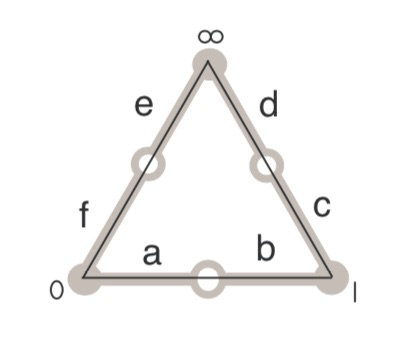But then, you may argue, this extender sends all of $0,1$ and $\infty$ to $0$, so it cannot belong to $\mathcal{E}_{MMM}$.

Here’s a trick to construct Belyi-extenders from Belyi-maps $\beta : \mathbb{P}^1 \rightarrow \mathbb{P}^1$, defined over $\mathbb{Q}$ and having the property that there are rational points in the fibers over $0,1$ and $\infty$.

Let’s take an example, the ‘monstrous dessin’ corresponding to the congruence subgroup $\Gamma_0(2)$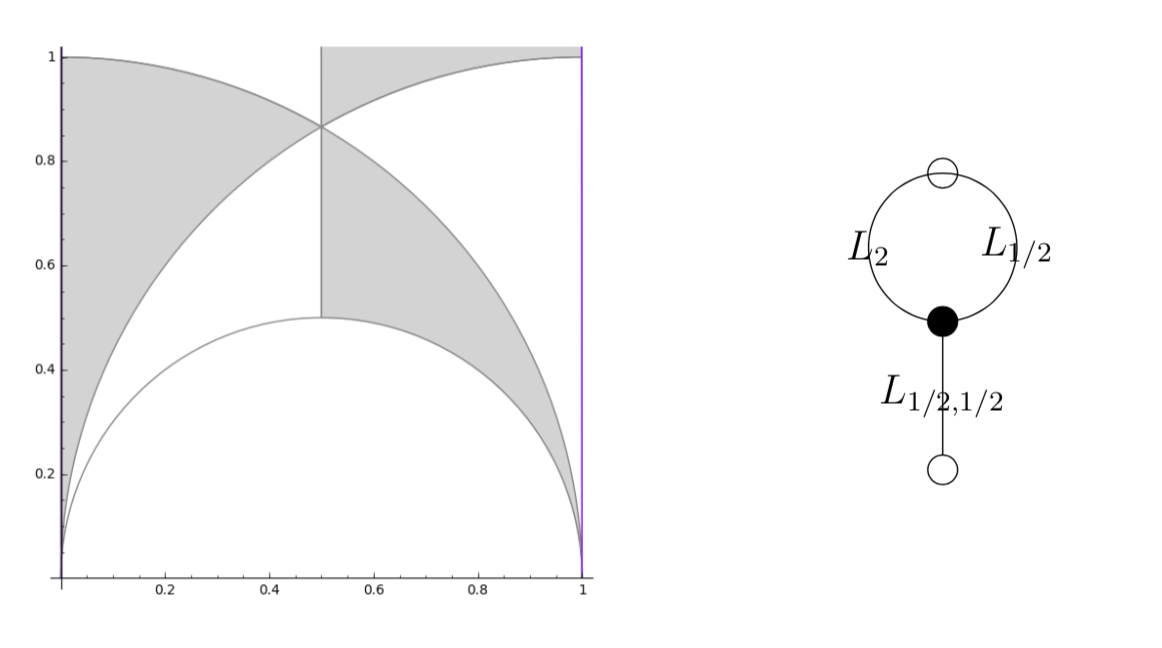with map $\beta(t) = \frac{(t+256)^3}{1728 t^2}$.

As it stands, $\beta$ is not a Belyi-extender because it does not map $1$ into $\{ 0,1,\infty \}$. But we have that
$-256 \in \beta^{-1}(0),~\infty \in \beta^{-1}(\infty),~\text{and}~512,-64 \in \beta^{-1}(1)$
(the last one follows from $(t+256)^2-1728 t^3=(t-512)^2(t+64)$).

We can now pre-compose $\beta$ with the automorphism (defined over $\mathbb{Q}$) sending $0$ to $-256$, $1$ to $-64$ and fixing $\infty$ to get a Belyi-extender
$\gamma(t) = \frac{(192t)^3}{1728(192t-256)^2}$
which maps $\gamma(0)=0,~\gamma(1)=1$ and $\gamma(\infty)=\infty$ (so belongs to $\mathcal{E}_{MMM}$) with the same dessin, which is not a tree,

That is, $\mathcal{E}_{MMM}$ can at best consist only of those Belyi-extenders $\gamma(t)$ that map $\{ 0,1,\infty \}$ onto $\{ 0,1,\infty \}$ and such that their dessin is a tree.

Let me stop, for now, by asking for a reference (or counterexample) to perhaps the most startling claim in the Manin-Marcolli paper, namely that any 2-coloured tree can be realised as the dessin of a Belyi-extender!

Dedekind’s Psi-function $\Psi(n)= n \prod_{p |n}(1 + \frac{1}{p})$ pops up in a number of topics:

• $\Psi(n)$ is the index of the congruence subgroup $\Gamma_0(n)$ in the modular group $\Gamma=PSL_2(\mathbb{Z})$,
• $\Psi(n)$ is the number of points in the projective line $\mathbb{P}^1(\mathbb{Z}/n\mathbb{Z})$,
• $\Psi(n)$ is the number of classes of $2$-dimensional lattices $L_{M \frac{g}{h}}$ at hyperdistance $n$ in Conway’s big picture from the standard lattice $L_1$,
• $\Psi(n)$ is the number of admissible maximal commuting sets of operators in the Pauli group of a single qudit.

The first and third interpretation have obvious connections with Monstrous Moonshine.

Conway’s big picture originated from the desire to better understand the Moonshine groups, and Ogg’s Jack Daniels problem
asks for a conceptual interpretation of the fact that the prime numbers such that $\Gamma_0(p)^+$ is a genus zero group are exactly the prime divisors of the order of the Monster simple group.

Here’s a nice talk by Ken Ono : Can’t you just feel the Moonshine?

For this reason it might be worthwhile to make the connection between these two concepts and the number of points of $\mathbb{P}^1(\mathbb{Z}/n\mathbb{Z})$ as explicit as possible.

Surely all of this is classical, but it is nicely summarised in the paper by Tatitscheff, He and McKay “Cusps, congruence groups and monstrous dessins”.

The ‘monstrous dessins’ from their title refers to the fact that the lattices $L_{M \frac{g}{h}}$ at hyperdistance $n$ from $L_1$ are permuted by the action of the modular groups and so determine a Grothendieck’s dessin d’enfant. In this paper they describe the dessins corresponding to the $15$ genus zero congruence subgroups $\Gamma_0(n)$, that is when $n=1,2,3,4,5,6,7,8,9,10,12,13,16,18$ or $25$.

Here’s the ‘monstrous dessin’ for $\Gamma_0(6)$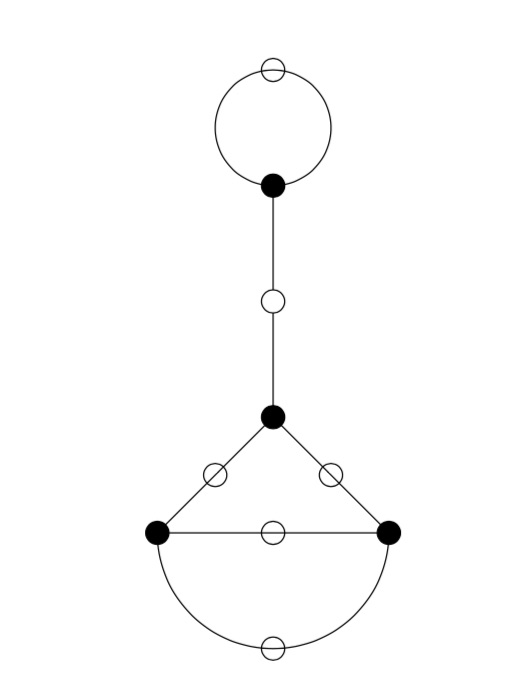But, one can compute these dessins for arbitrary $n$, describing the ripples in Conway’s big picture, and try to figure out whether they are consistent with the Riemann hypothesis.

We will get there eventually, but let’s start at an easy pace and try to describe the points of the projective line $\mathbb{P}^1(\mathbb{Z}/n \mathbb{Z})$.

Over a field $k$ the points of $\mathbb{P}^1(k)$ correspond to the lines through the origin in the affine plane $\mathbb{A}^2(k)$ and they can represented by projective coordinates $[a:b]$ which are equivalence classes of couples $(a,b) \in k^2- \{ (0,0) \}$ under scalar multiplication with non-zero elements in $k$, so with points $[a:1]$ for all $a \in k$ together with the point at infinity $[1:0]$. When $n=p$ is a prime number we have $\# \mathbb{P}^1(\mathbb{Z}/p\mathbb{Z}) = p+1$. Here are the $8$ lines through the origin in $\mathbb{A}^2(\mathbb{Z}/7\mathbb{Z})$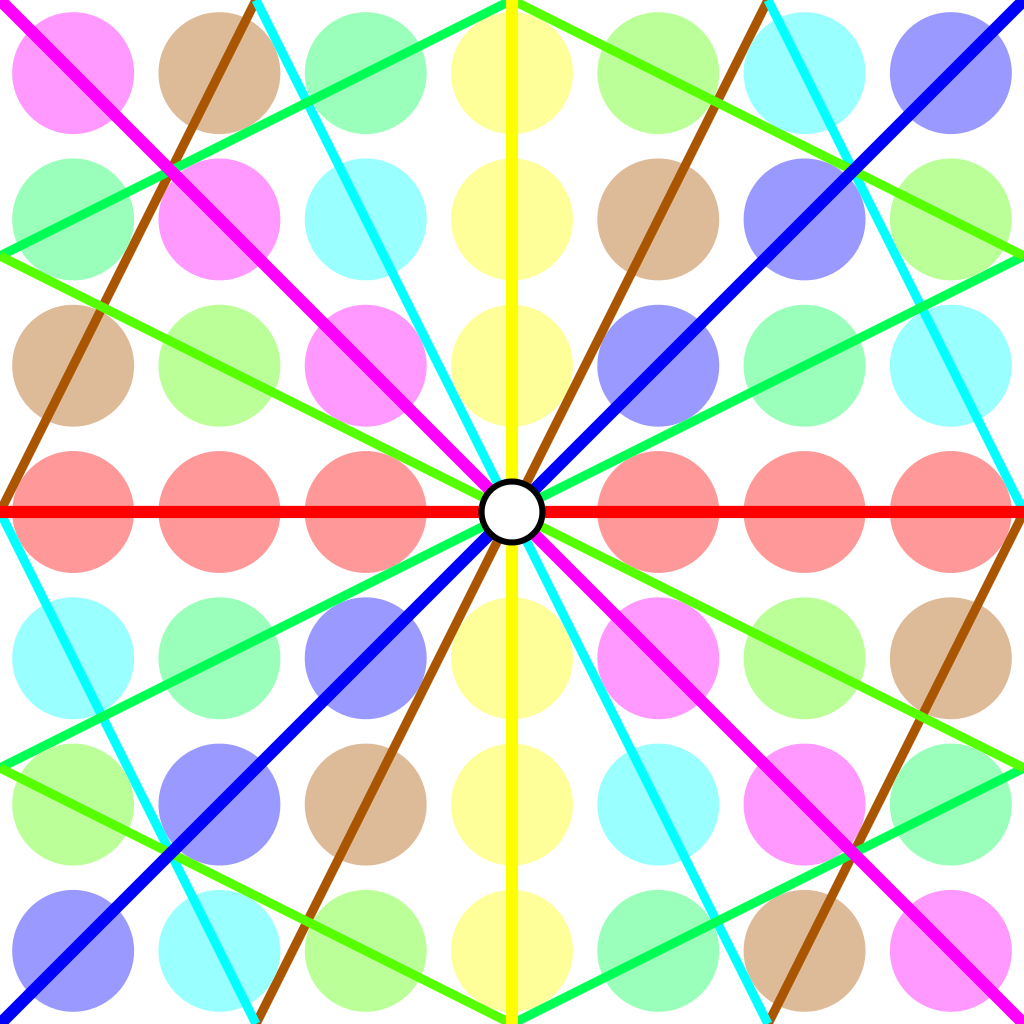Over an arbitrary (commutative) ring $R$ the points of $\mathbb{P}^1(R)$ again represent equivalence classes, this time of pairs
$(a,b) \in R^2~:~aR+bR=R$
with respect to scalar multiplication by units in $R$, that is
$(a,b) \sim (c,d)~\quad~\text{iff}~\qquad \exists \lambda \in R^*~:~a=\lambda c, b = \lambda d$
For $\mathbb{P}^1(\mathbb{Z}/n \mathbb{Z})$ we have to find all pairs of integers $(a,b) \in \mathbb{Z}^2$ with $0 \leq a,b < n$ with $gcd(a,b)=1$ and use Cremona’s trick to test for equivalence:
$(a,b) = (c,d) \in \mathbb{P}^1(\mathbb{Z}/n \mathbb{Z})~\quad \text{iff}~\quad ad-bc \equiv 0~mod~n$
The problem is to find a canonical representative in each class in an efficient way because this is used a huge number of times in working with modular symbols.

Perhaps the best algorithm, for large $n$, is sketched in pages 145-146 of Bill Stein’s Modular forms: a computational approach.

For small $n$ the algorithm in $\S 1.3$ in the Tatitscheff, He and McKay paper suffices:

• Consider the action of $(\mathbb{Z}/n\mathbb{Z})^*$ on $\{ 0,1,…,n-1 \}=\mathbb{Z}/n\mathbb{Z}$ and let $D$ be the set of the smallest elements in each orbit,
• For each $d \in D$ compute the stabilizer subgroup $G_d$ for this action and let $C_d$ be the set of smallest elements in each $G_d$-orbit on the set of all elements in $\mathbb{Z}/n \mathbb{Z}$ coprime with $d$,
• Then $\mathbb{P}^1(\mathbb{Z}/n\mathbb{Z})= \{ [c:d]~|~d \in D, c \in C_d \}$.

Let’s work this out for $n=12$ which will be our running example (the smallest non-squarefree non-primepower):

• $(\mathbb{Z}/12\mathbb{Z})^* = \{ 1,5,7,11 \} \simeq C_2 \times C_2$,
• The orbits on $\{ 0,1,…,11 \}$ are
$\{ 0 \}, \{ 1,5,7,11 \}, \{ 2,10 \}, \{ 3,9 \}, \{ 4,8 \}, \{ 6 \}$
and $D=\{ 0,1,2,3,4,6 \}$,
• $G_0 = C_2 \times C_2$, $G_1 = \{ 1 \}$, $G_2 = \{ 1,7 \}$, $G_3 = \{ 1,5 \}$, $G_4=\{ 1,7 \}$ and $G_6=C_2 \times C_2$,
• $1$ is the only number coprime with $0$, giving us $[1:0]$,
• $\{ 0,1,…,11 \}$ are all coprime with $1$, and we have trivial stabilizer, giving us the points $[0:1],[1:1],…,[11:1]$,
• $\{ 1,3,5,7,9,11 \}$ are coprime with $2$ and under the action of $\{ 1,7 \}$ they split into the orbits
$\{ 1,7 \},~\{ 3,9 \},~\{ 5,11 \}$
giving us the points $[1:2],[3:2]$ and $[5:2]$,
• $\{ 1,2,4,5,7,8,10,11 \}$ are coprime with $3$, the action of $\{ 1,5 \}$ gives us the orbits
$\{ 1,5 \},~\{ 2,10 \},~\{ 4,8 \},~\{ 7,11 \}$
and additional points $[1:3],[2:3],[4:3]$ and $[7:3]$,
• $\{ 1,3,5,7,9,11 \}$ are coprime with $4$ and under the action of $\{ 1,7 \}$ we get orbits
$\{ 1,7 \},~\{ 3,9 \},~\{ 5,11 \}$
and points $[1:4],[3:4]$ and $[5,4]$,
• Finally, $\{ 1,5,7,11 \}$ are the only coprimes with $6$ and they form a single orbit under $C_2 \times C_2$ giving us just one additional point $[1:6]$.

This gives us all $24= \Psi(12)$ points of $\mathbb{P}^1(\mathbb{Z}/12 \mathbb{Z})$ (strangely, op page 43 of the T-H-M paper they use different representants).

One way to see that $\# \mathbb{P}^1(\mathbb{Z}/n \mathbb{Z}) = \Psi(n)$ comes from a consequence of the Chinese Remainder Theorem that for the prime factorization $n = p_1^{e_1} … p_k^{e_k}$ we have
$\mathbb{P}^1(\mathbb{Z}/n \mathbb{Z}) = \mathbb{P}^1(\mathbb{Z}/p_1^{e_1} \mathbb{Z}) \times … \times \mathbb{P}^1(\mathbb{Z}/p_k^{e_k} \mathbb{Z})$
and for a prime power $p^k$ we have canonical representants for $\mathbb{P}^1(\mathbb{Z}/p^k \mathbb{Z})$
$[a:1]~\text{for}~a=0,1,…,p^k-1~\quad \text{and} \quad [1:b]~\text{for}~b=0,p,2p,3p,…,p^k-p$
which shows that $\# \mathbb{P}^1(\mathbb{Z}/p^k \mathbb{Z}) = (p+1)p^{k-1}= \Psi(p^k)$.

Next time, we’ll connect $\mathbb{P}^1(\mathbb{Z}/n \mathbb{Z})$ to Conway’s big picture and the congruence subgroup $\Gamma_0(n)$.

Last time we revisited Robin’s theorem saying that 5040 being the largest counterexample to the bound
$\frac{\sigma(n)}{n~log(log(n))} < e^{\gamma} = 1.78107...$ is equivalent to the Riemann hypothesis.

There’s an industry of similar results using other arithmetic functions. Today, we’ll focus on Dedekind’s Psi function
$\Psi(n) = n \prod_{p | n}(1 + \frac{1}{p})$
where $p$ runs over the prime divisors of $n$. It is series A001615 in the online encyclopedia of integer sequences and it starts off with

1, 3, 4, 6, 6, 12, 8, 12, 12, 18, 12, 24, 14, 24, 24, 24, 18, 36, 20, 36, 32, 36, 24, 48, 30, 42, 36, 48, 30, 72, 32, 48, 48, 54, 48, …

and here’s a plot of its first 1000 values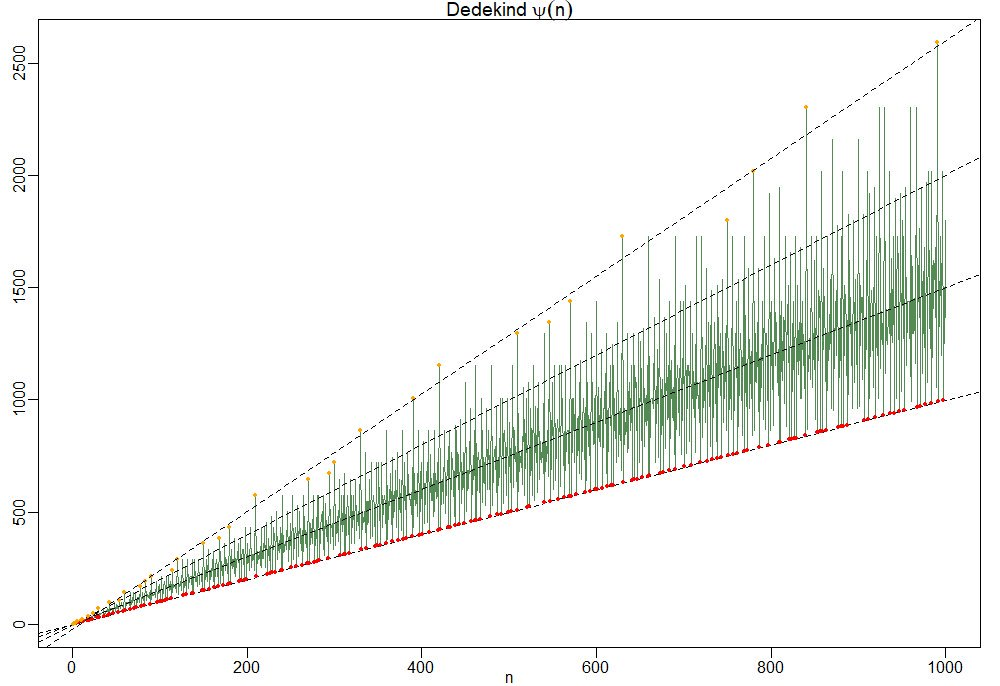To understand this behaviour it is best to focus on the ‘slopes’ $\frac{\Psi(n)}{n}=\prod_{p|n}(1+\frac{1}{p})$.

So, the red dots of minimal ‘slope’ $\approx 1$ correspond to the prime numbers, and the ‘outliers’ have a maximal number of distinct small prime divisors. Look at $210 = 2 \times 3 \times 5 \times 7$ and its multiples $420,630$ and $840$ in the picture.

For this reason the primorial numbers, which are the products of the fist $k$ prime numbers, play a special role. This is series A002110 starting off with

1, 2, 6, 30, 210, 2310, 30030, 510510, 9699690, 223092870,…

In Patrick Solé and Michel Planat Extreme values of the Dedekind $\Psi$ function, it is shown that the primorials play a similar role for Dedekind’s Psi as the superabundant numbers play for the sum-of-divisors function $\sigma(n)$.

That is, if $N_k$ is the $k$-th primorial, then for all $n < N_k$ we have that the 'slope' at $n$ is strictly below that of $N_k$ $\frac{\Psi(n)}{n} < \frac{\Psi(N_k)}{N_k}$ which follows immediately from the fact that any $n < N_k$ can have at most $k-1$ distinct prime factors and $p \mapsto 1 + \frac{1}{p}$ is a strictly decreasing function.

Another easy, but nice, observation is that for all $n$ we have the inequalities
$n^2 > \phi(n) \times \psi(n) > \frac{n^2}{\zeta(2)}$
where $\phi(n)$ is Euler’s totient function
$\phi(n) = n \prod_{p | n}(1 – \frac{1}{p})$
This follows as once from the definitions of $\phi(n)$ and $\Psi(n)$
$\phi(n) \times \Psi(n) = n^2 \prod_{p|n}(1 – \frac{1}{p^2}) < n^2 \prod_{p~\text{prime}} (1 - \frac{1}{p^2}) = \frac{n^2}{\zeta(2)}$ But now it starts getting interesting.

In the proof of his theorem, Guy Robin used a result of his Ph.D. advisor Jean-Louis Nicolas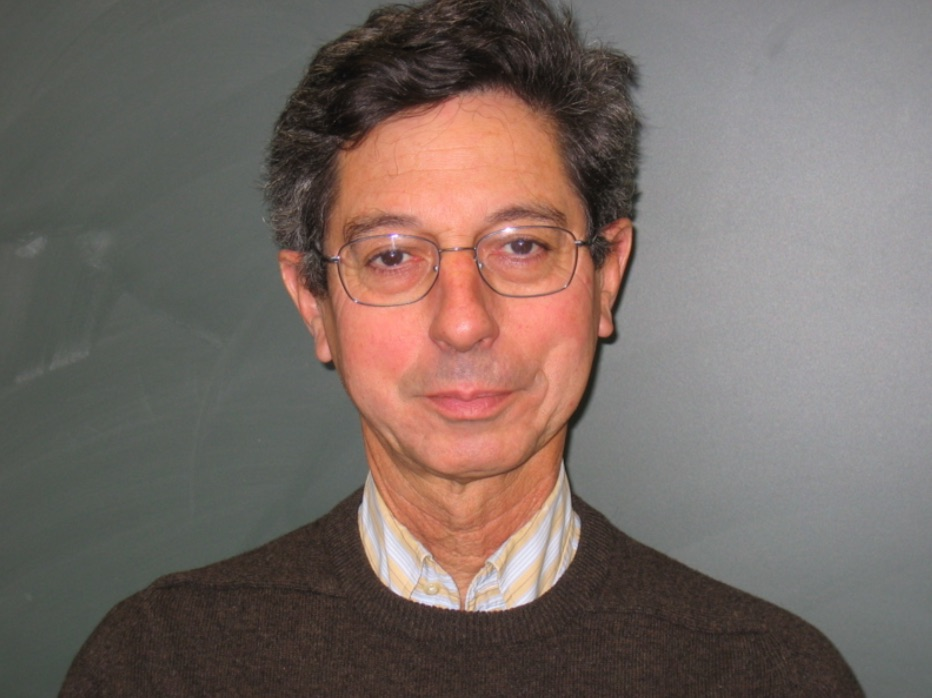known as Nicolas’ criterion for the Riemann hypothesis: RH is true if and only if for all $k$ we have the inequality for the $k$-th primorial number $N_k$
$\frac{N_k}{\phi(N_k)~log(log(N_k))} > e^{\gamma}$
From the above lower bound on $\phi(n) \times \Psi(n)$ we have for $n=N_k$ that
$\frac{\Psi(N_k)}{N_k} > \frac{N_k}{\phi(N_k) \zeta(2)}$
and combining this with Nicolas’ criterion we get
$\frac{\Psi(N_k)}{N_k~log(log(N_k))} > \frac{N_k}{\phi(N_k)~log(log(N_k)) \zeta(2)} > \frac{e^{\gamma}}{\zeta(2)} \approx 1.08…$
In fact, Patrick Solé and Michel Planat prove in their paper Extreme values of the Dedekind $\Psi$ function that RH is equivalent to the lower bound
$\frac{\Psi(N_k)}{N_k~log(log(N_k))} > \frac{e^{\gamma}}{\zeta(2)}$
holding for all $k \geq 3$.

Dedekind’s Psi function pops up in lots of interesting mathematics.

In the theory of modular forms, Dedekind himself used it to describe the index of the congruence subgroup $\Gamma_0(n)$ in the full modular group $\Gamma$.

In other words, it gives us the number of tiles needed in the Dedekind tessellation to describe the fundamental domain of the action of $\Gamma_0(n)$ on the upper half-plane by Moebius transformations.When $n=6$ we have $\Psi(6)=12$ and we can view its fundamental domain via these Sage commands:

 G=Gamma0(6) FareySymbol(G).fundamental_domain() 

giving us the 24 back or white tiles (note that these tiles are each fundamental domains of the extended modular group, so we have twice as many of them as for subgroups of the modular group)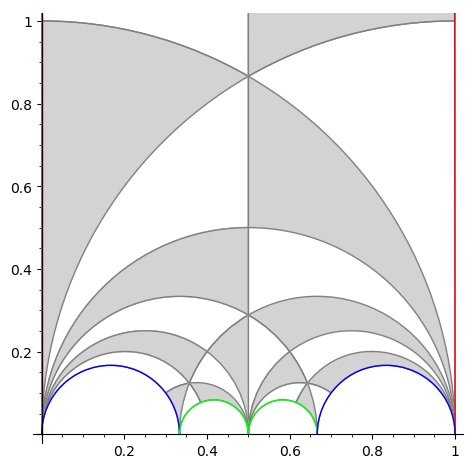But, there are plenty of other, seemingly unrelated, topics where $\Psi(n)$ appears. To name just a few:

• The number of points on the projective line $\mathbb{P}^1(\mathbb{Z}/n\mathbb{Z})$.
• The number of lattices at hyperdistance $n$ in Conway’s big picture.
• The number of admissible maximal commuting sets of operators in the Pauli group for the $n$ qudit.

and there are explicit natural one-to-one correspondences between all these manifestations of $\Psi(n)$, tbc.

“She simply walked into the pond in Kensington Gardens Sunday morning and drowned herself in three feet of water.”

This is the opening sentence of The Ishango Bone, a novel by Paul Hastings Wilson. It (re)tells the story of a young mathematician at Cambridge, Amiele, who (dis)proves the Riemann Hypothesis at the age of 26, is denied the Fields medal, and commits suicide.

In his review of the novel on MathFiction, Alex Kasman casts he story in the 1970ties, based on the admission of the first female students to Trinity.

More likely, the correct time frame is in the first decade of this century. On page 121 Amiele meets Alain Connes, said to be a “past winner of the Crafoord Prize”, which Alain obtained in 2001. In fact, noncommutative geometry and its interaction with quantum physics plays a crucial role in her ‘proof’.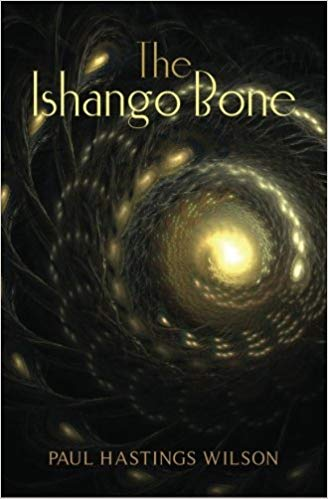The Ishango artefact only appears in the Coda to the book. There are a number of theories on the nature and grouping of the scorings on the bone. In one column some people recognise the numbers 11, 13, 17 and 19 (the primes between 10 and 20).

In the book, Amiele remarks that the total number of lines scored on the bone (168) “happened to be the exact total of all the primes between 1 and 1000” and “if she multiplied 60, the total number of lines in one side column, by 168, the grand total of lines, she’d get 10080,…,not such a far guess from 9592, the actual total of primes between 1 and 100000.” (page 139-140)

The bone is believed to be more than 20000 years old, prime numbers were probably not understood until about 500 BC…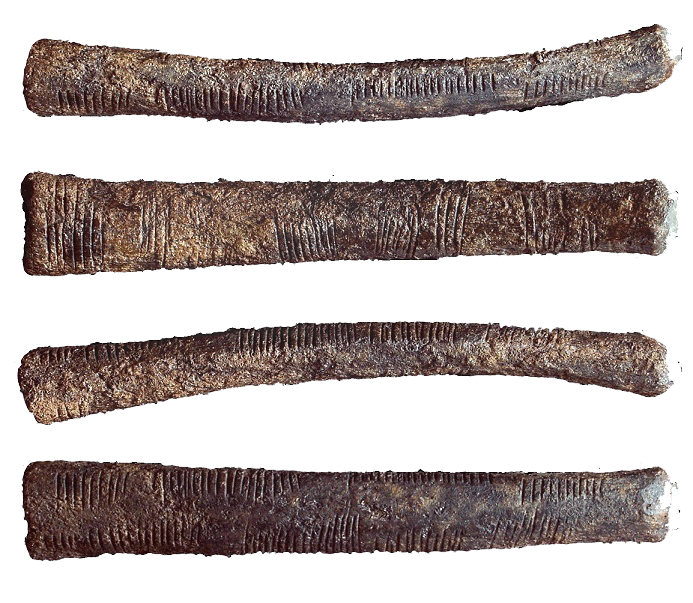More interesting than these speculations on the nature of the Ishango bone is the description of the tools Amiele thinks to need to tackle the Riemann Hypothesis:

“These included algebraic geometry (which combines commutative algebra with the language and problems of geometry); noncommutative geometry (concerned with the geometric approach to associative algebras, in which multiplication is not commutative, that is, for which $x$ times $y$ does not always equal $y$ times $x$); quantum field theory on noncommutative spacetime, and mathematical aspects of quantum models of consciousness, to name a few.” (page 115)

The breakthrough came two years later when Amiele was giving a lecture on Grothendieck’s dessins d’enfant.

“Dessin d’enfant, or ‘child’s drawing’, which Amiele had discovered in Grothendieck’s work, is a type of graph drawing that seemed technically simple, but had a very strong impression on her, partly due to the familiar nature of the objects considered. (…) Amiele found subtle arithmetic invariants associated with these dessins, which were completely transformed, again, as soon as another stroke was added.” (page 116)

Amiele’s ‘disproof’ of RH is outlined on pages 122-124 of “The Ishango Bone” and is a mixture of recognisable concepts and ill-defined terms.

“Her final result proved that Riemann’s Hypothesis was false, a zero must fall to the east of Riemann’s critical line whenever the zeta function of point $q$ with momentum $p$ approached the aelotropic state-vector (this is a simplification, of course).” (page 123)

More details are given in a footnote:

“(…) a zero must fall to the east of Riemann’s critical line whenever:

$\zeta(q_p) = \frac{( | \uparrow \rangle + \Psi) + \frac{1}{2}(1+cos(\Theta))\frac{\hbar}{\pi}}{\int(\Delta_p)}$

(…) The intrepid are invited to try the equation for themselves.” (page 124)

Wilson’s “The Ishango Bone” was published in 2012. A fair number of topics covered (the Ishango bone, dessin d’enfant, Riemann hypothesis, quantum theory) also play a prominent role in the 2015 paper/story by Michel Planat “A moonshine dialogue in mathematical physics”, but this time with additional story-line: monstrous moonshine

Such a paper surely deserves a separate post.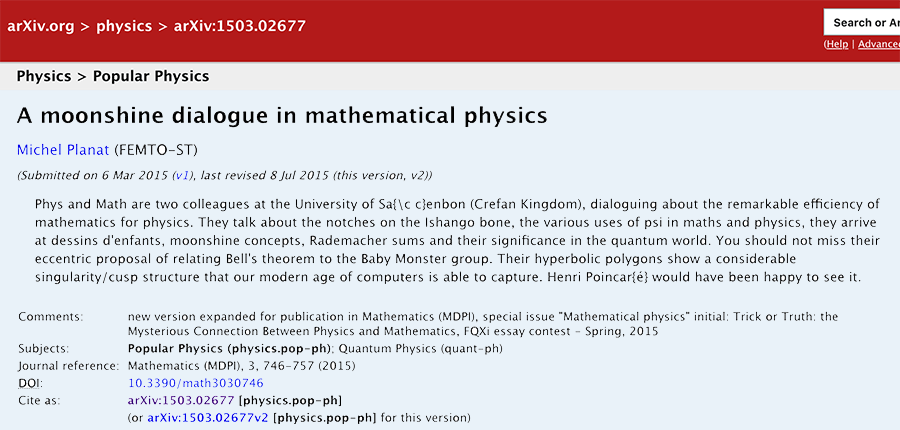‘Gabriel’s topos’ (see here) is the conjectural, but still elusive topos from which the validity of the Riemann hypothesis would follow.

It is the latest attempt in Alain Connes’ 20 year long quest to tackle the RH (before, he tried the tools of noncommutative geometry and later those offered by the field with one element).

For the last 5 years he hopes that topos theory might provide the missing ingredient. Together with Katia Consani he introduced and studied the geometry of the Arithmetic site, and later the geometry of the scaling site.

If you look at the points of these toposes you get horribly complicated ‘non-commutative’ spaces, such as the finite adele classes $\mathbb{Q}^*_+ \backslash \mathbb{A}^f_{\mathbb{Q}} / \widehat{\mathbb{Z}}^{\ast}$ (in case of the arithmetic site) and the full adele classes $\mathbb{Q}^*_+ \backslash \mathbb{A}_{\mathbb{Q}} / \widehat{\mathbb{Z}}^{\ast}$ (for the scaling site).

In Vienna, Connes gave a nice introduction to the arithmetic site in two lectures. The first part of the talk below also gives an historic overview of his work on the RH

The second lecture can be watched here.

However, not everyone is as optimistic about the topos-approach as he seems to be. Here’s an insightful answer on MathOverflow by Will Sawin to the question “What is precisely still missing in Connes’ approach to RH?”.

Other interesting MathOverflow threads related to the RH-approach via the field with one element are Approaches to Riemann hypothesis using methods outside number theory and Riemann hypothesis via absolute geometry.

About a month ago, from May 10th till 14th Alain Connes gave a series of lectures at Ohio State University with title “The Riemann-Roch strategy, quantizing the Scaling Site”.

The accompanying paper has now been arXived: The Riemann-Roch strategy, Complex lift of the Scaling Site (joint with K. Consani).

Especially interesting is section 2 “The geometry behind the zeros of $\zeta$” in which they explain how looking at the zeros locus inevitably leads to the space of adele classes and why one has to study this space with the tools from noncommutative geometry.

Perhaps further developments will be disclosed in a few weeks time when Connes is one of the speakers at Toposes in Como.No kidding, this is the final sentence of Le spectre d’Atacama, the second novel by Alain Connes (written with Danye Chéreau (IRL Mrs. AC) and his former Ph.D. advisor Jacques Dixmier).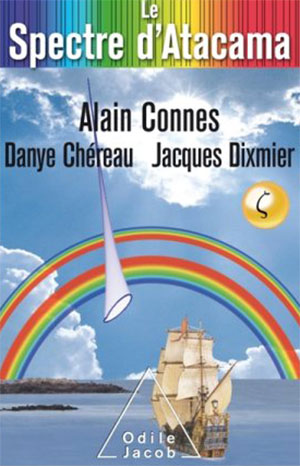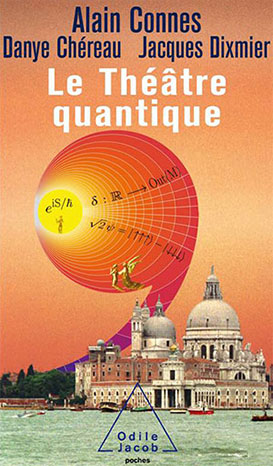The book has a promising start. Armand Lafforet (IRL AC) is summoned by his friend Rodrigo to the Chilean observatory Alma in the Altacama desert. They have observed a mysterious spectrum, and need his advice.

Armand drops everything and on the flight he lectures the lady sitting next to him on proofs by induction (breaking up chocolate bars), and recalls a recent stay at the La Trappe Abbey, where he had an encounter with (the ghost of) Alexander Grothendieck, who urged him to ‘Follow the motif!’.

“Comment était-il arrivé là? Il possédait surement quelques clés. Pourquoi pas celles des songes?” (How did he get
there? Surely he owned some keys, why not those of our dreams?)

A few pages further there’s this on the notion of topos (my attempt to translate):

“The notion of space plays a central role in mathematics. Traditionally we represent it as a set of points, together with a notion of neighborhood that we call a ‘topology’. The universe of these new spaces, ‘toposes’, unveiled by Grothendieck, is marvellous, not only for the infinite wealth of examples (it contains, apart from the ordinary topological spaces, also numerous instances of a more combinatorial nature) but because of the totally original way to perceive space: instead of appearing on the main stage from the start, it hides backstage and manifests itself as a ‘deus ex machina’, introducing a variability in the theory of sets.”

So far, so good.

We have a mystery, tidbits of mathematics, and allusions left there to put a smile on any Grothendieck-aficionado’s face.

But then, upon arrival, the story drops dead.

Rodrigo has been taken to hospital, and will remain incommunicado until well in the final quarter of the book.

As the remaining astronomers show little interest in Alain’s (sorry, Armand’s) first lecture, he decides to skip the second, and departs on a hike to the ocean. There, he takes a genuine sailing ship in true Jules Verne style to the lighthouse at he end of the world.

All this drags on for at least half a year in time, and two thirds of the book’s length. We are left in complete suspense when it comes to the mysterious Atacama spectrum.

Perhaps the three authors deliberately want to break with existing conventions of story telling?

I had a similar feeling when reading their first novel Le Theatre Quantique. Here they spend some effort to flesh out their heroine, Charlotte, in the first part of the book. But then, all of a sudden, their main character is replaced by a detective, and next by a computer.

Anyway, when Armand finally reappears at the IHES the story picks up pace.

The trio (Armand, his would-be-lover Charlotte, and Ali Ravi, Cern’s computer guru) convince CERN to sell its main computer to an American billionaire with the (fake) promise of developing a quantum computer. Incidentally, they somehow manage to do this using Charlotte’s history with that computer (for this, you have to read ‘Le Theatre Quantique’).

By their quantum-computing power (Shor and quantum-encryption pass the revue) they are able to decipher the Atacame spectrum (something to do with primes and zeroes of the zeta function), send coded messages using quantum entanglement, end up in the Oval Office and convince the president to send a message to the ‘Riemann sphere’ (another fun pun), and so on, and on.

The book ends with a twist of the classic tale of the mathematician willing to sell his soul to the devil for a (dis)proof of the Riemann hypothesis:

After spending some time in purgatory, the mathematician gets a meeting with God and asks her the question “Is the Riemann hypothesis true?”.

“Of course”, God says.

“But how can you know that all non-trivial zeroes of the zeta function have real part 1/2?”, Armand asks.

And God replies:

“Simple enough, I can see them all at once. But then, don’t forget I’m God. I can see the disappointment in your face, yes I can read in your heart that you are frustrated, that you desire an explanation…

Well, we’re going to fix this. I will call archangel Gabriel, the angel of geometry, he will make you a topos!”

If you feel like running to the nearest Kindle store to buy “Le spectre d’Atacama”, make sure to opt for a package deal. It is impossible to make heads or tails of the story without reading “Le theatre quantique” first.

But then, there are worse ways to spend an idle week than by binge reading Connes…

Edit (February 28th). A short video of Alain Connes explaining ‘Le spectre d’Atacama’ (in French)

The Bulletin of the AMS just made this paper by Julia Mueller available online: “On the genesis of Robert P. Langlands’ conjectures and his letter to Andre Weil” (hat tip +ChandanDalawat and +DavidRoberts on Google+).

It recounts the story of the early years of Langlands and the first years of his mathematical career (1960-1966)leading up to his letter to Andre Weil in which he outlines his conjectures, which would become known as the Langlands program.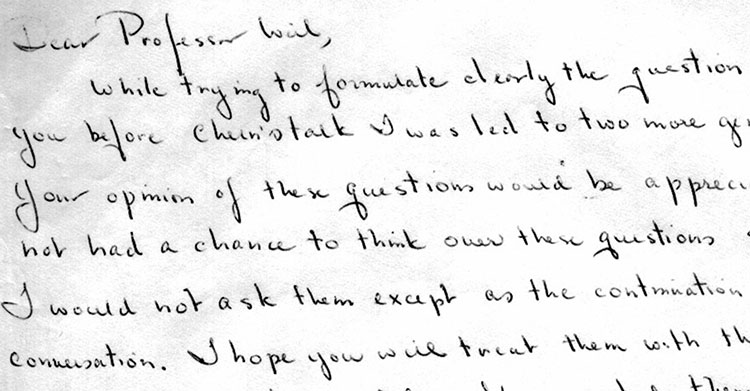Langlands letter to Weil is available from the IAS.

The Langlands program is a vast net of conjectures. For example, it conjectures that there is a correspondence between

– $n$-dimensional representations of the absolute Galois group $Gal(\overline{\mathbb{Q}}/\mathbb{Q})$, and

– specific data coming from an adelic quotient-space $GL_n(\mathbb{A}_{\mathbb{Q}})/GL_n(\mathbb{Q})$.

For $n=1$ this is essentially class field theory with the correspondence given by Artin’s reciprocity law.

Here we have on the one hand the characters of the abelianised absolute Galois group

$Gal(\overline{\mathbb{Q}}/\mathbb{Q})^{ab} \simeq Gal(\mathbb{Q}(\pmb{\mu}_{\infty})/\mathbb{Q}) \simeq \widehat{\mathbb{Z}}^{\ast}$

and on the other hand the connected components of the idele class space

$GL_1(\mathbb{A}_{\mathbb{Q}})/GL_1(\mathbb{Q}) = \mathbb{A}_{\mathbb{Q}}^{\ast} / \mathbb{Q}^{\ast} = \mathbb{R}_+^{\ast} \times \widehat{\mathbb{Z}}^{\ast}$

For $n=2$ it involves the study of Galois representations coming from elliptic curves. A gentle introduction to the general case is Mark Kisin’s paper What is … a Galois representation?.

One way to look at some of the quantum statistical systems studied via non-commutative geometry is that they try to understand the “bad” boundary of the Langlands space $GL_n(\mathbb{A}_{\mathbb{Q}})/GL_n(\mathbb{Q})$.

Here, the Bost-Connes system corresponds to the $n=1$ case, the Connes-Marcolli system to the $n=2$ case.

If $\mathbb{A}’_{\mathbb{Q}}$ is the subset of all adeles having almost all of its terms in $\widehat{\mathbb{Z}}_p^{\ast}$, then there is a well-defined map

$\pi~:~\mathbb{A}’_{\mathbb{Q}}/\mathbb{Q}^{\ast} \rightarrow \mathbb{R}_+ \qquad (x_{\infty},x_2,x_2,\dots) \mapsto | x_{\infty} | \prod_p | x_p |_p$

The inverse image of $\pi$ over $\mathbb{R}_+^{\ast}$ are exactly the idele classes $\mathbb{A}_{\mathbb{Q}}^{\ast}/\mathbb{Q}^{\ast}$, so we can view them as the nice locus of the horrible complicated quotient of adele-classes $\mathbb{A}_{\mathbb{Q}}/\mathbb{Q}^*$. And we can view the adele-classes as a ‘closure’ of the idele classes.

But, the fiber $\pi^{-1}(0)$ has horrible topological properties because $\mathbb{Q}^*$ acts ergodically on it due to the fact that $log(p)/log(q)$ is irrational for distinct primes $p$ and $q$.

This is why it is better to view the adele-classes not as an ordinary space (one with bad topological properties), but rather as a ‘non-commutative’ space because it is controlled by a non-commutative algebra, the Bost-Connes algebra.

For $n=2$ there’s a similar story with a ‘bad’ quotient $M_2(\mathbb{A}_{\mathbb{Q}})/GL_2(\mathbb{Q})$, being the closure of an ‘open’ nice piece which is the Langlands quotient space $GL_2(\mathbb{A}_{\mathbb{Q}})/GL_2(\mathbb{Q})$.